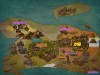# 星星灰暗着。# 星星灰暗着。

### ARC不会做选讲

posted on 2018-11-18 08:36:00 | under Summary |

## $061F$

$n+m+k\le 300000$ 。

$UPD:$ 好像有简单的 $O(n)DP$ ，是我沙雕了。

## $087E$

$Alice$ 先手，请问谁必胜。
$L\le 10^{18},\vert S\vert\le 10^5,s_1+\cdots+s_n\le 10^5,s_i$ 表示初始第 $i$ 个串长度。

$$sg(x)=\mathrm{mex}(0,sg(x-1),sg(x-1)\oplus sg(x-2),\cdots,sg(x-1)\oplus\cdots sg(1))$$

## $093E$

$n\le 10^3,m\le 2\times 10^3$ 。

$val=x$ ，那么这条边可以任意涂，除了所有这种情况的边的生成树的颜色都是一样的情况。
$val<x$ ，这条边一定得和其它这种情况的边是同一种颜色，并且得和这些生成树上的边是同一种颜色。那么它们的颜色是被绑定的，不算就可以了。

## $096E$

$600pts:n\le 50$
$900pts:n\le 3000$

$$ans=\sum_{i=0}^{n}(-1)^i2^{2^{n-i}}\binom{n}{i}\sum_{j=0}^{i}\binom{ i}{j}\sum_{k=1}^{j}\begin{Bmatrix}j\\k\end{Bmatrix}(2^{n-i})^k$$

## $097E$

$n\le2000$ 。

## $100D$

$n\le 2\times 10^5,a_i\le 10^9$ 。